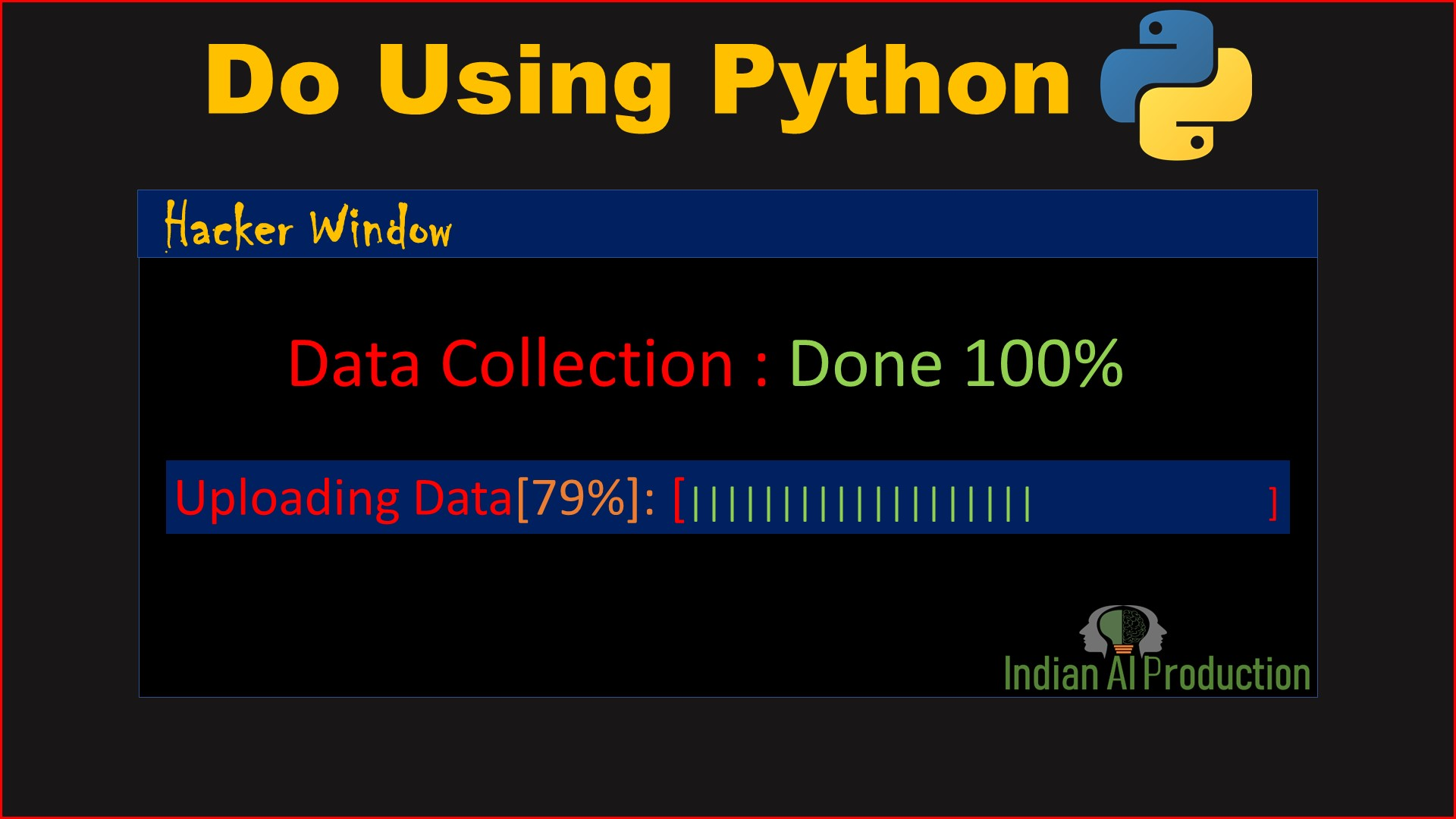## Change the Pixel Value of an Image in OpenCV Python | OpenCV Tutorial

In Python OpenCV Tutorial, Explained How to Change Pixels Color in an image using NumPy Slicing and indexing. Get the answers of below questions: What is pixel?What is resolution?How to read image pixel?How to get image pixel?How to print image pixel?How to change the pixel value of an image in python opencv?How do I pixelate an image in OpenCV?How do I change the pixel value of an image in...## Get Image Pixels Using OpenCV Python | OpenCV Tutorial in Hindi

In Python OpenCV Tutorial, Explained How to get image pixel using NumPy Slicing and indexing. How to access image pixels Below code executed on Jupyter Notebook # Import Library import cv2 import numpy as np # Read & Show Image img_path = r"C:\Users\kashz\AI Life\AI Projects - IAIP, PTs (Web + Channel)\02 OpenCV\000 opencv tutorial\data\pexels-rafi-ahmed-haven-1253364.jpg" img =...## How to Dilate(Dilation) Image using OpenCV Python

Morphological Operations Morphological transformations are some simple operations based on the image shape. It is normally performed on binary images. It needs two inputs, one is our original image, the second one is called structuring element or kernel which decides the nature of the operation. Two basic morphological operators are Erosion and Dilation. Then its variant forms like Opening,...## How to Erode(Erosion) Image using OpenCV Python

Morphological Operations Morphological transformations are some simple operations based on the image shape. It is normally performed on binary images. It needs two inputs, one is our original image, the second one is called structuring element or kernel which decides the nature of the operation. Two basic morphological operators are Erosion and Dilation. Then its variant forms like Opening,...## Create Zeros vs Zeros _like vs Zeros _initializer Tensor Using TensorFlow 2.0 Python Tutorial

In TensorFlow 2.0, we can create Zeros Tensor(every element in tensor are zeros) in different ways using tf.zeros() and tf.zeros_like() and tf.zeros_initializer(). Create Zeros Tesnor using tf.zeros() Creates a tensor with all elements set to zero (0) Syntax: tf.zeros(shape, dtype=tf.dtypes.float32, name=None) -> Return ZerosTensor """ Create Zeros vs Zeros _like vs Zeros _initializer...## Create Ones vs Ones_like vs Ones_initializer Tensor Using TensorFlow 2.0 Python Tutorial

In TensorFlow 2.0, we can create Ones Tensor(every element in tensor are ones) in different ways using tf.ones() and tf.ones_like() and tf.ones_initializer(). Create Ones Tesnor using tf.ones() Creates a tensor with all elements set to one (1) Syntax: tf.ones(shape, dtype=tf.dtypes.float32, name=None) -> Return Ones Tensor """ Create Ones vs Ones_like vs Ones_initializer Tensor Using TensorFlow...## Create Sparse Tensor using TensorFlow 2.0 Python Tutorial

What is Sparse Tensor? Tensor that contain mostly zero values are called sparse tensor. When working with tensors that contain a lot of zero values, it is important to store them in a space- and time-efficient manner. Sparse tensors enable efficient storage and processing of tensors that contain a lot of zero values.Sparse tensors are used extensively in encoding schemes like TF-IDF as part of...## Create TensorFlow Placeholder using TensorFlow 2.X Python Tutorial

A TensorFlow placeholder is simply a variable that we will assign data to at a later date. It allows us to create our operations and build our computation graph, without needing the data. Syntax: tf.compat.v1.placeholder(dtype, shape=None, name=None) How to Use TensorFlow Placeholder In TensorFlow 2.X How Migrate your TensorFlow 1 code to TensorFlow 2 """ Video: Create TensorFlow Placeholder...## How to Create variable in TensorFlow 2.x Tutorial

The Variable() constructor requires an initial value for the variable, which can be a Tensor of any type and shape. This initial value defines the type and shape of the variable. After construction, the type and shape of the variable are fixed. The value can be changed using one of the assign methods. Syntax: tf.Variable(initial_value=None, trainable=None, validate_shape=True,...## How print colorful text in Python | Python Tutorial

In Python Tutorial in Hindi, We will Learn how print colorful text in Python using TermColor Library. In this video tutorial going to cover: How do you type in color text?What Colour is a string in Python?How do you change text color in idle Python?What are the colors in python?How do you make text bold in Python? Termcolor : ANSII Color formatting for output in terminal. Termcolor...
Top